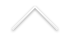Título: Fast mixing time for the exclusion process in a random environment

Palestrante: Hubert Lacoin (IMPA)
Data: 07/06/2021
Horário: 15:00hrs
Local: Transmissão online

Confira AQUI o link para a transmissão.

Resumo: We consider a system of $k$ particles on a segment of size $N$. Its rules of evolution are the following: a particle at site $x \in \{1 ,\dots, N\}$ jumps to $x+1$ with rate $\omega_x$ and to $x-1$ with rate $1-\omega_x$ where $\omega_x\in (0,1)$, and a jump is cancelled if the site is already occupied. We consider the case where $(\omega_x)^N_{x=1}$ is (the fixed realization of) a sequence of IID random variables. Assuming that $\mathbb E[ \log\frac{1-\omega_x}{\omega_x}]\ne 0$ (that is, transience of the random environment), we prove that this particle systems mixes fast, in the sense that the time that it requires for its distribution to get close to the equilibrium state grows like a power of $N$. We present a lower bound for the power in mixing time which we conjecture to be sharp. Joint work with S. Yang, IMPA.

Acesse AQUI as gravações dos seminários online de 2020.

Este ano, alguns dias depois da reunião, os vídeos devem estar disponíveis AQUI.

Todas as palestras são ministradas em inglês.